# How much would you have to Invest today to receive the following? Use Appendix B and Appendix D for an approximate ans...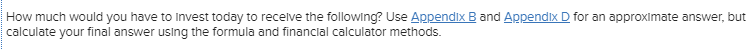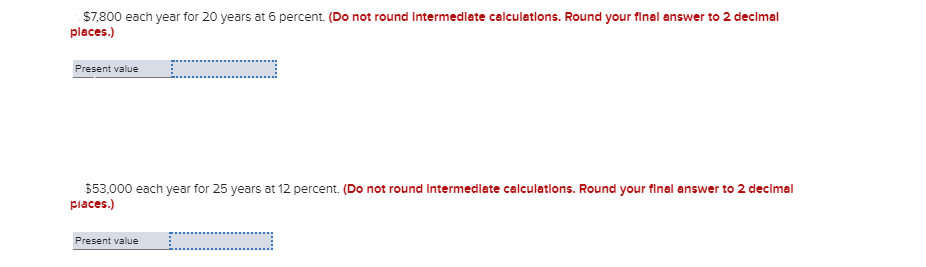How much would you have to Invest today to receive the following? Use Appendix B and Appendix D for an approximate answer, but calculate your final answer using the formula and financial calculator methods.
\$7,800 each year for 20 years at 6 percent. (Do not round Intermediate calculations. Round your final answer to 2 decimal places.) Present value 553,000 each year for 25 years at 12 percent. (Do not round Intermediate calculations. Round your final answer to 2 decimal places.) Present value Present value D .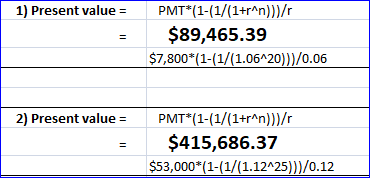#### Earn Coin

Coins can be redeemed for fabulous gifts.

Similar Homework Help Questions
• ### How much would you have to invest today to receive the following? Use Appendix B or...

How much would you have to invest today to receive the following? Use Appendix B or Appendix D for an approximate answer, but calculate your final answer using the formula and financial calculator methods.    a. \$15,250 in 11 years at 7 percent. (Do not round intermediate calculations. Round your final answer to 2 decimal places.)    b. \$19,600 in 18 years at 11 percent. (Do not round intermediate calculations. Round your final answer to 2 decimal places.)    c....

• ### If you invest \$9,000 today, how much will you have in each of the following instances?...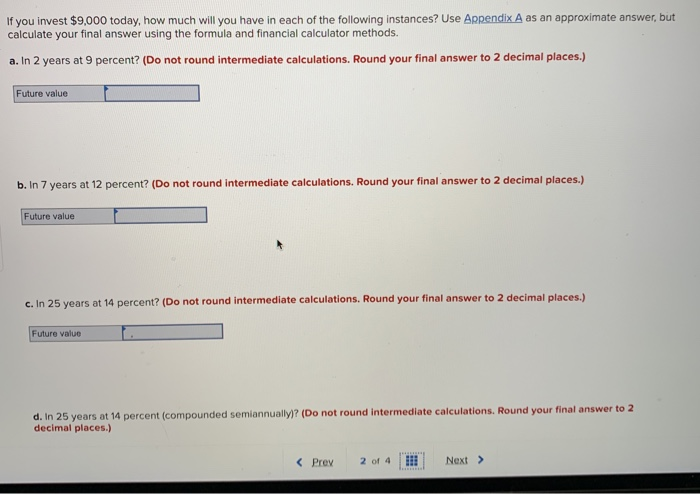If you invest \$9,000 today, how much will you have in each of the following instances? Use Appendix A as an approximate answer, but calculate your final answer using the formula and financial calculator methods a. In 2 years at 9 percent? (Do not round intermediate calculations. Round your final answer to 2 decimal places.) Future value b. In 7 years at 12 percent? (Do not round intermediate calculations. Round your final answer to 2 decimal places.) Future value c....

• ### What is the present value of the following? Use Appendix B as an approximate answer, but...

What is the present value of the following? Use Appendix B as an approximate answer, but calculate your final answer using the formula and financial calculator methods. a. \$7,800 in 6 years at 10 percent? (Do not round intermediate calculations. Round your final answer to 2 decimal places.)    b. \$16,500 in 3 years at 7 percent? (Do not round intermediate calculations. Round your final answer to 2 decimal places.)    c. \$25,700 in 9 years at 8 percent? (Do...

• ### What is the present value of the following? Use Appendix B as an approximate answer, but...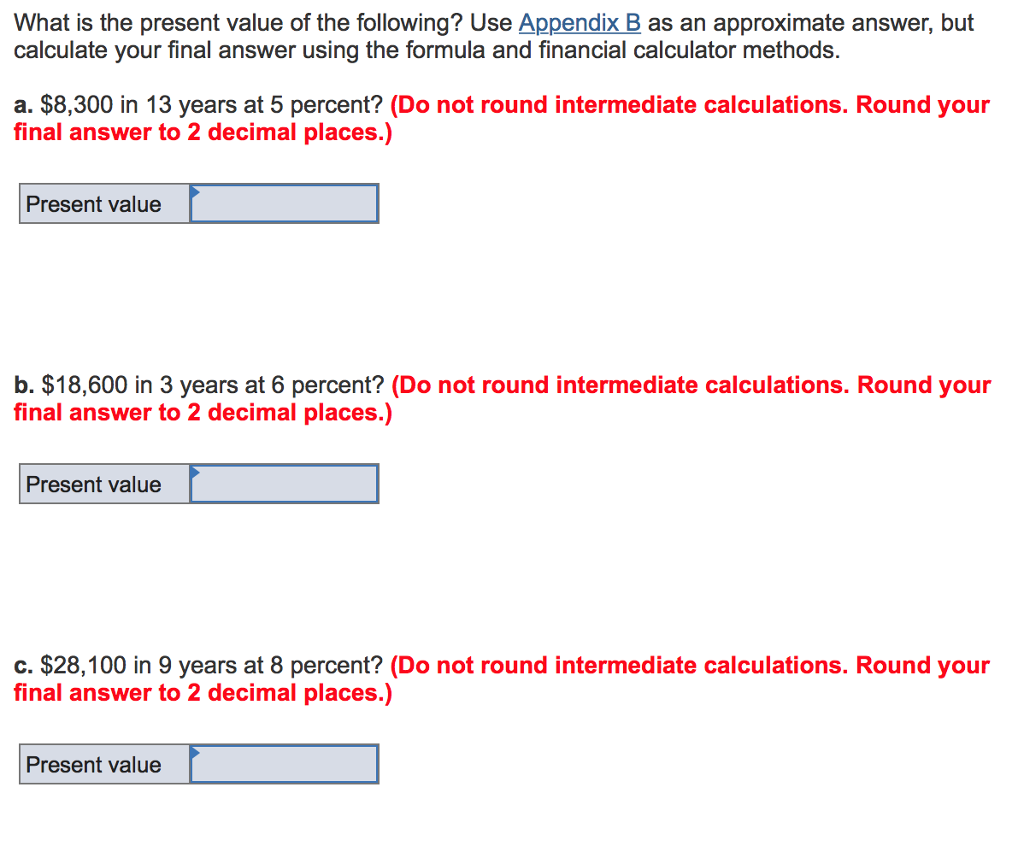What is the present value of the following? Use Appendix B as an approximate answer, but calculate your final answer using the formula and financial calculator methods. a. \$8,300 in 13 years at 5 percent? (Do not round intermediate calculations. Round your final answer to 2 decimal places.) Present value b. \$18,600 in 3 years at 6 percent? (Do not round intermediate calculations. Round your final answer to 2 decimal places.) Present value c. \$28,100 in 9 years at 8...

• ### What is the present value of the following? Use Appendix B as an approximate answer, but...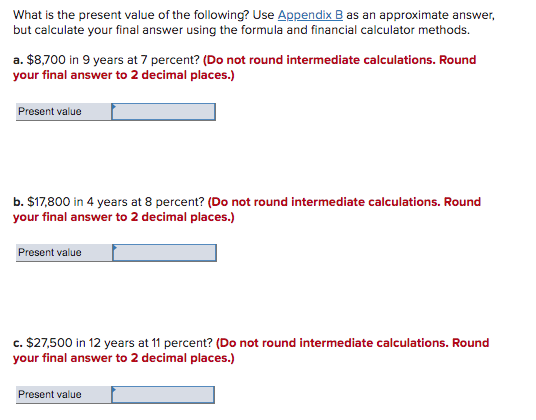What is the present value of the following? Use Appendix B as an approximate answer, but calculate your final answer using the formula and financial calculator methods. a. \$8,700 in 9 years at 7 percent? (Do not round intermediate calculations. Round your final answer to 2 decimal places.) Present value b. \$17,800 in 4 years at 8 percent? (Do not round intermediate calculations. Round your final answer to 2 decimal places.) Present value C. \$27,500 in 12 years at 11...

• ### What is the present value of the following? Use Appendix B as an approximate answer, but...

What is the present value of the following? Use Appendix B as an approximate answer, but calculate your final answer using the formula and financial calculator methods. a. \$8,600 in 5 years at 10 percent? (Do not round intermediate calculations. Round your final answer to 2 decimal places.)    b. \$17,600 in 3 years at 6 percent? (Do not round intermediate calculations. Round your final answer to 2 decimal places.) c. \$27,100 in 9 years at 7 percent? (Do not...

• ### How much would you have to invest today to receive the following ? Calculate answer using...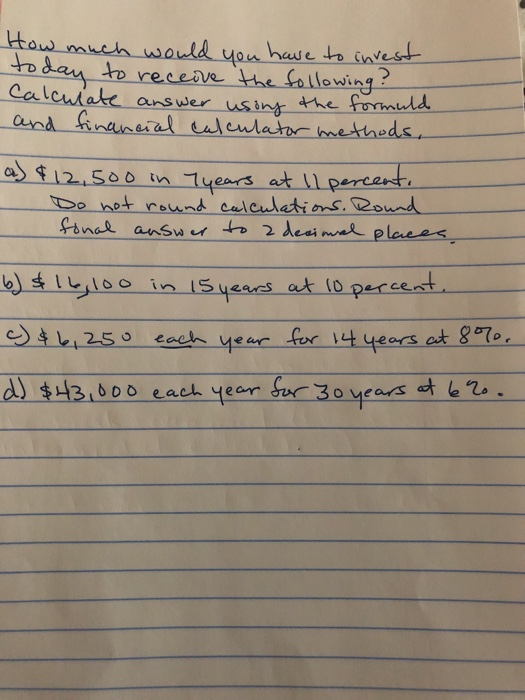How much would you have to invest today to receive the following ? Calculate answer using the formuld and financial calculator methods, a) 4 12,500 in 7 years at 11 percent. Do not round calculations. Round fonal answer to a decimal places b) \$ 16, 100 in 15 years at 10 percent. c) & 6, 250 each year for 14 years at 8%. d) \$43,000 each year for 30 years at 6ro.

• ### If you invest \$8,200 per period for the following number of periods, how much would you...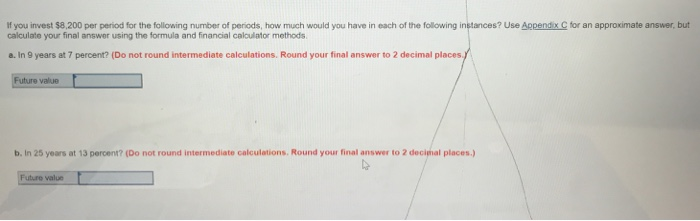If you invest \$8,200 per period for the following number of periods, how much would you have in each of the following instances? Use Appendix C for an approximate answer, but calculate your final answer using the formula and financial calculator methods a. In 9 years at 7 percent? (Do not round intermediate calculations. Round your final answer to 2 decimal places.) Future value b. In 25 years at 13 percent? (Do not round intermediate calculations. Round your final answer...

• ### Your grandfather has offered you a choice of one of the three following alternatives: \$5,500 now; \$1,250 a year for five years; or \$17,000 at the end of five years. Use Appendix B and Appendix D for an approximate answer, but calculate your final answer u

Your grandfather has offered you a choice of one of the three following alternatives: \$5,500 now; \$1,250 a year for five years; or \$17,000 at the end of five years. Use Appendix B and Appendix D for an approximate answer, but calculate your final answer using the formula and financial calculator methods.a-1. Assuming you could earn 6 percent annually, compute the present value of each alternative: (Do not round intermediate calculations. Round your final answers to 2 decimal places.)1250 ->    Present value = _________  a-2. If you...

• ### If you invest \$9,400 per period for the following number of periods, how much would you...

If you invest \$9,400 per period for the following number of periods, how much would you have in each of the following instances? Use Appendix C for an approximate answer, but calculate your final answer using the formula and financial calculator methods. a. In 12 years at 9 percent? (Do not round intermediate calculations. Round your final answer to 2 decimal places.)In 25 years at 9 percent? (Do not round intermediate calculations. Round your final answer to 2 decimal places.)